# Approximation of a generalized additive mapping in multi-Banach modules and isomorphisms in multi-$C ∗$-algebras: a fixed-point approach

## Abstract

Let $X$, $Y$ be vector spaces. It is shown that if an odd mapping $f:X→Y$ satisfies the functional equation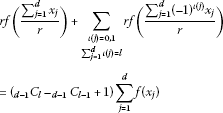(0.1)

then the odd mapping $f:X→Y$ is additive, and we use a fixed-point method to prove the Hyers-Ulam stability of the functional equation (0.1) in multi-Banach modules over a unital multi-$C ∗$-algebra. As an application, we show that every almost linear bijection $h:A→B$ of a unital multi-$C ∗$-algebra $A$ onto a unital multi-$C ∗$-algebra $B$ is a $C ∗$-algebra isomorphism when $h( 2 n r n uy)=h( 2 n r n u)h(y)$ for all unitaries $u∈U(A)$, all $y∈A$, and $n=0,1,2,…$ .

MSC:39B52, 46L05, 47H10, 47B48.

## 1 Introduction

Throughout this paper we assume that r is a positive rational number and d, l are integers with $1.

Let X and Y be Banach spaces. Consider a mapping $f:X→Y$ such that $f(tx)$ is continuous in $t∈R$ for each fixed $x∈X$, and assume that there exist constants $θ≥0$ and $p∈[0,1)$ with

$∥ f ( x + y ) − f ( x ) − f ( y ) ∥ ≤θ ( ∥ x ∥ p + ∥ y ∥ p ) ,x,y∈X.$

Rassias  showed that there exists a unique $R$-linear mapping $T:X→Y$ such that

$∥ f ( x ) − T ( x ) ∥ ≤ 2 θ 2 − 2 p ∥ x ∥ p ,x∈X.$

Găvruta  extended the above theorem as follows: let G be an Abelian group, Y be a Banach space and put

$φ ˜ (x,y)= ∑ j = 0 ∞ 1 2 j φ ( 2 j x , 2 j y ) <∞,x,y∈G.$

If $f:G→Y$ is a mapping satisfying

$∥ f ( x + y ) − f ( x ) − f ( y ) ∥ ≤φ(x,y),x,y∈G,$

then there exists a unique additive mapping $T:G→Y$ such that

$∥ f ( x ) − T ( x ) ∥ ≤ 1 2 φ ˜ (x,x),x∈G.$

Park  applied Găvruta’s result to linear functional equations in Banach modules over a $C ∗$-algebra. Several functional equations have been investigated in [4, 5] and . In 2006 Baak, Boo and Rassias  solved the following functional equation: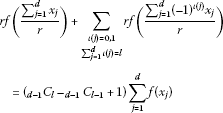(1.1)

(any solution of (1.1) will be called a generalized additive mapping) and proved its Hyers-Ulam stability in Banach modules over a unital $C ∗$-algebra via the direct method. These results were applied to investigate $C ∗$-algebra isomorphisms in unital $C ∗$-algebras.

In this paper, we prove the Hyers-Ulam stability of the functional equation (1.1) in multi-Banach modules over a unital multi-$C ∗$-algebra via the fixed-point method. These results are applied to investigate $C ∗$-algebra isomorphisms in unital multi-$C ∗$-algebras.

## 2 Fixed-point theorems

We recall two fundamental results in the fixed-point theory.

Theorem 2.1 [8, 9]

Let $(X,d)$ be a complete metric space and let $J:X→X$ be strictly contractive, i.e.,

$d(Jx,Jy)≤Ld(x,y),x,y∈X$

for a Lipschitz constant $L<1$. Then

1. (1)

the mapping J has a unique fixed point $x ∗ ∈X$,

2. (2)

the fixed point $x ∗$ is globally attractive, i.e.,

$lim n → ∞ J n x= x ∗ ,x∈X,$
3. (3)

the following inequalities hold:

$d ( J n x , x ∗ ) ≤ L n d ( x , x ∗ ) , d ( J n x , x ∗ ) ≤ 1 1 − L d ( J n x , J n + 1 x ) , d ( x , x ∗ ) ≤ 1 1 − L d ( x , J x )$

for all $x∈X$ and nonnegative integers n.

Let X be a non-empty set. A function $d:X×X→[0,∞]$ is called a generalized metric on X if for any $x,y,z∈X$, we have:

1. (1)

$d(x,y)=0$ if and only if $x=y$,

2. (2)

$d(x,y)=d(y,x)$,

3. (3)

$d(x,z)≤d(x,y)+d(y,z)$.

Theorem 2.2 [8, 10]

Let $(X,d)$ be a complete generalized metric space and let $J:X→X$ be a strictly contractive mapping with a Lipschitz constant $L<1$. Then, for each $x∈X$, either

$d ( J n x , J n + 1 x ) =∞,n≥0$

or there exists a positive integer $n 0$ such that:

1. (1)

$d( J n x, J n + 1 x)<∞$, $n≥ n 0$,

2. (2)

the sequence $( J n x)$ converges to a fixed point $y ∗$ of J,

3. (3)

$y ∗$ is the unique fixed point of J in the set $Y={y∈X:d( J n 0 x,y)<∞}$,

4. (4)

$d(y, y ∗ )≤ 1 1 − L d(y,Jy)$, $y∈Y$.

## 3 Multi-normed spaces

The notion of a multi-normed space was introduced by Dales and Polyakov . This concept is somewhat similar to an operator sequence space and has some connections with operator spaces and Banach lattices. Motivations for the study of multi-normed spaces and many examples are given in .

Let $(E,∥⋅∥)$ be a complex normed space and $k∈N$. We denote by $E k$ the linear space $E⊕⋯⊕E$ consisting of k-tuples $( x 1 ,…, x k )$, where $x 1 ,…, x k ∈E$. The linear operations on $E k$ are defined coordinate-wise. The zero element of either $E$ or $E k$ is denoted by 0. Finally, we denote by $N k$ the set ${1,…,k}$ and by $Σ k$ the group of permutations on k symbols.

Definition 3.1 [11, 14] A multi-norm on ${ E k :k∈N}$ is a sequence

$( ∥ ⋅ ∥ k ) = ( ∥ ⋅ ∥ k : k ∈ N )$

such that $∥ ⋅ ∥ k$ is a norm on $E k$ for $k∈N$, $∥ ⋅ ∥ 1 =∥⋅∥$, and for any integer $k≥2$, we have

(A1) $∥ ( x σ ( 1 ) , … , x σ ( k ) ) ∥ k = ∥ ( x 1 , … , x k ) ∥ k$, $σ∈ Σ k$, $x 1 ,…, x k ∈E$,

(A2) $∥ ( α 1 x 1 , … , α k x k ) ∥ k ≤( max i ∈ N k | α i |) ∥ ( x 1 , … , x k ) ∥ k$, $α 1 ,…, α k ∈C$, $x 1 ,…, x k ∈E$,

(A3) $∥ ( x 1 , … , x k − 1 , 0 ) ∥ k = ∥ ( x 1 , … , x k − 1 ) ∥ k − 1$, $x 1 ,…, x k − 1 ∈E$,

(A4) $∥ ( x 1 , … , x k − 1 , x k − 1 ) ∥ k = ∥ ( x 1 , … , x k − 1 ) ∥ k − 1$, $x 1 ,…, x k − 1 ∈E$.

A sequence $(( E k , ∥ ⋅ ∥ k ):k∈N)$ is then said to be a multi-normed space.

Lemma 3.2 [11, 13]

Suppose that $(( E k , ∥ ⋅ ∥ k ):k∈N)$ is a multi-normed space. Then for any $k∈N$, we have

1. (a)

$∥ ( x , … , x ) ∥ k =∥x∥$, $x∈E$,

2. (b)

$max i ∈ N k ∥ x i ∥≤ ∥ ( x 1 , … , x k ) ∥ k ≤ ∑ i = 1 k ∥ x i ∥≤k max i ∈ N k ∥ x i ∥$, $x 1 ,…, x k ∈E$.

From Lemma 3.2(b), it follows that if $(E,∥⋅∥)$ is a Banach space, then $( E k , ∥ ⋅ ∥ k )$ is a Banach space for each $k∈N$ (in this case we say that $(( E k , ∥ ⋅ ∥ k ):k∈N)$ is a multi-Banach space).

Now, we recall two important examples of multi-norms (see [11, 12]).

Example 3.3 The sequence $( ∥ ⋅ ∥ k :k∈N)$ on ${ E k :k∈N}$ defined by

$∥ ( x 1 , … , x k ) ∥ k := max i ∈ N k ∥ x i ∥, x 1 ,…, x k ∈E$

is a multi-norm called the minimum multi-norm. The terminology ‘minimum’ is justified by property (b) from Lemma 3.2.

Example 3.4 Let ${( ∥ ⋅ ∥ k α :k∈N):α∈A}$ be a (non-empty) family of all multi-norms on ${ E k :k∈N}$. For $k∈N$, set

$⦀( x 1 ,…, x k ) ⦀ k := sup α ∈ A ∥ ( x 1 , … , x k ) ∥ k α , x 1 ,…, x k ∈E.$

Then $(⦀⋅ ⦀ k :k∈N)$ is a multi-norm on ${ E k :k∈N}$ called the maximum multi-norm.

Lemma 3.5 

Suppose that $k∈N$ and $( x 1 ,…, x k )∈ E k$. For each $j∈{1,…,k}$, let $( x n j ) n ∈ N$ be a sequence in $E$ such that $lim n → ∞ x n j = x j$. Then for each $( y 1 ,…, y k )∈ E k$ we have

$lim n → ∞ ( x n 1 − y 1 , … , x n k − y k ) =( x 1 − y 1 ,…, x k − y k ).$

Definition 3.6 [12, 14]

Let $(( E k , ∥ ⋅ ∥ k ):k∈N)$ be a multi-normed space. A sequence $( x n ) n ∈ N$ in $E$ is said to be a multi-null sequence if for each $ε>0$, there exists an $n 0 ∈N$ such that

$sup k ∈ N ∥ ( x n , … , x n + k − 1 ) ∥ k <ε,n≥ n 0 .$

We say that the sequence $( x n ) n ∈ N$ is multi-convergent to $x∈E$ and write $lim n → ∞ x n =x$ if $( x n − x ) n ∈ N$ is a multi-null sequence.

Definition 3.7 [11, 14]

Let $(A,∥⋅∥)$ be a normed algebra such that $(( A k , ∥ ⋅ ∥ k ):k∈N)$ is a multi-normed space. Then $(( A k , ∥ ⋅ ∥ k ):k∈N)$ is called a multi-normed algebra if

$∥ ( a 1 b 1 , … , a k b k ) ∥ k ≤ ∥ ( a 1 , … , a k ) ∥ k ⋅ ∥ ( b 1 , … , b k ) ∥ k ,k∈N, a 1 ,…, a k , b 1 ,…, b k ∈A.$

The multi-normed algebra $(( A k , ∥ ⋅ ∥ k ):k∈N)$ is said to be a multi-Banach algebra if $(( A k , ∥ ⋅ ∥ k ):k∈N)$ is a multi-Banach space.

Example 3.8 Let p, q be such that $1≤p≤q<∞$ and $A= ℓ p$. The algebra $A$ is a Banach sequence algebra with respect to coordinate-wise multiplication of sequences (see Example 4.1.42 of ). Let $( ∥ ⋅ ∥ k :k∈N)$ be the standard $(p,q)$-multi-norm on ${ A k :k∈N}$ (see ). Then $(( A k , ∥ ⋅ ∥ k ):k∈N)$ is a multi-Banach algebra.

Definition 3.9 Let $(( A k , ∥ ⋅ ∥ k ):k∈N)$ be a multi-Banach algebra and assume that $A$ is a (unital) $C ∗$-algebra. If the involution satisfies

$∥ ( a 1 ∗ a 1 , … , a k ∗ a k ) ∥ k = ∥ ( a 1 , … , a k ) ∥ k 2 ,k∈N, a 1 ,…, a k ∈A,$

then $(( A k , ∥ ⋅ ∥ k ):k∈N)$ is called a (unital) multi-$C ∗$-algebra.

Definition 3.10 Let $(( A k , ∥ ⋅ ∥ k ):k∈N)$ be a multi-Banach algebra and $(( X k , ∥ ⋅ ∥ k ):k∈N)$ be a multi-Banach space. Assume also that $X$ is a Banach left module over $A$. We say that $(( X k , ∥ ⋅ ∥ k ):k∈N)$ is a multi-Banach left module over $(( A k , ∥ ⋅ ∥ k ):k∈N)$ if there is an $M≥0$ such that

$∥ ( a 1 x 1 , … , a k x k ) ∥ k ≤M ∥ ( a 1 , … , a k ) ∥ k ⋅ ∥ ( x 1 , … , x k ) ∥ k$

for all $k∈N$, $a 1 ,…, a k ∈A$, $x 1 ,…, x k ∈X$.

## 4 Stability of an odd functional equation in multi-Banach modules over a multi-$C ∗$-algebra

Throughout this section, we assume that $(( A k , ∥ ⋅ ∥ k ):k∈N)$ is a unital multi-$C ∗$-algebra, and $(( X k , ∥ ⋅ ∥ k ):k∈N)$ and $(( Y k , ∥ ⋅ ∥ k ):k∈N)$ are multi-Banach left modules over $(( A k , ∥ ⋅ ∥ k ):k∈N)$. Moreover, by $U(A)$ we denote the unitary group of $A$.

Lemma 4.1 

Let X and Y be vector spaces. An odd mapping $f:X→Y$ satisfies (1.1) for all $x 1 ,…, x d ∈X$ if and only if f is additive.

Corollary 4.2 

Let X and Y be vector spaces. An odd mapping $f:X→Y$ satisfies

$rf ( x + y r ) =f(x)+f(y),x,y∈X$

if and only if f is additive.

Given a mapping $f:X→Y$, we set

$D u f ( x 1 , … , x d ) : = r f ( ∑ j = 1 d u x j r ) + ∑ ι ( j ) = 0 , 1 ∑ j = 1 d ι ( j ) = l r f ( ∑ j = 1 d ( − 1 ) ι ( j ) u x j r ) − ( d − 1 C l − d − 1 C l − 1 + 1 ) ∑ j = 1 d u f ( x j )$

for all $u∈U(A)$ and $x 1 ,…, x d ∈X$.

Theorem 4.3 Let $r≠2$ and $f:X→Y$ be an odd mapping such that for every $k∈N$ there is a function $φ k : X k d →[0,∞)$ with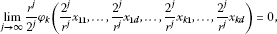(4.1)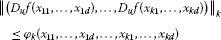(4.2)

for all $u∈U(A)$ and $x 11 ,…, x 1 d ,…, x k 1 ,…, x k d ∈X$. If there exists an $L<1$ such that

$φ k ( 2 r x 11 , 2 r x 11 , … , 0 ⏞ d , … , 2 r x k 1 , 2 r x k 1 , … , 0 ⏞ d ) ≤ 2 r L φ k ( x 11 , x 11 , … , 0 ⏞ d , … , x k 1 , x k 1 , … , 0 ⏞ d )$

for all $k∈N$ and $x 11 ,…, x k 1 ∈X$, then there is a unique $A$-linear generalized additive mapping $L:X→Y$ with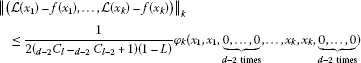(4.3)

for all $k∈N$ and $x 1 ,…, x k ∈X$.

Proof Put

$X:={L:X→Y}$

and

$d ( L , h ) = inf { C ∈ R + : ∥ ( L ( x 1 ) − h ( x 1 ) , … , L ( x k ) − h ( x k ) ) ∥ k ≤ C φ k ( x 1 , x 1 , 0 , … , 0 ⏞ d , … , x k , x k , 0 , … , 0 ⏞ d ) , k ∈ N , x 1 , … , x k ∈ X }$

for all $L,h∈X$. It is easy to show that $(X,d)$ is a complete generalized metric space.

Define a mapping $J:X→X$ by

$JL(x):= r 2 L ( 2 r x ) ,L∈X,x∈X.$

Analysis similar to that in the proof of Theorem 3.1 in  (see also the proof of Lemma 3.2 in ) shows that

$d(JL,Jh)≤Ld(L,h),L,h∈X.$

Fix $k∈N$. Putting $u=1∈U(A)$, $x i 1 = x i 2 = x 1$, and $x i 3 =⋯= x i d =0$ for $i∈{1,…,k}$ in (4.2), we have

$∥ ( r f ( 2 r x 1 ) − 2 f ( x 1 ) , … , r f ( 2 r x k ) − 2 f ( x k ) ) ∥ k ≤ 1 C l d − 2 − d − 2 C l − 2 + 1 φ k ( x 1 , x 1 , 0 , … , 0 ⏞ d − 2 times , … , x k , x k , 0 , … , 0 ⏞ d − 2 times ) ,$

because f is odd and $t: = d − 2 C l − d − 2 C l − 2 +1 = d − 1 C l − d − 1 C l − 1 +1$. We thus get

$∥ ( f ( x 1 ) − r 2 f ( 2 r x 1 ) , … , f ( x k ) − r 2 f ( 2 r x k ) ) ∥ k ≤ 1 2 t φ k ( x 1 , x 1 , 0 , … , 0 ⏞ d − 2 times , … , x k , x k , 0 , … , 0 ⏞ d − 2 times ) , x 1 , … , x k ∈ X ,$

and therefore,

$d(f,Jf)≤ 1 2 t .$
(4.4)

Consequently, by Theorem 2.2, there exists a mapping $L:X→Y$ such that

1. (1)

$L$ is a fixed point of J, i.e.,

$L ( 2 r x ) = 2 r L(x),x∈X,$
(4.5)

and $L$ is unique in the set

$Y= { L ∈ X : d ( f , L ) < ∞ } .$

This means that $L$ is a unique mapping satisfying (4.5) such that there exists a $C∈(0,∞)$ with

$∥ ( L ( x 1 ) − f ( x 1 ) , … , L ( x k ) − f ( x k ) ) ∥ k ≤C φ k ( x 1 , x 1 , 0 , … , 0 ⏞ d − 2 times ,…, x k , x k , 0 , … , 0 ⏞ d − 2 times )$

for all $k∈N$ and $x 1 ,…, x k ∈X$.

1. (2)

$d( J n f,L)→0$ as $n→∞$. This implies the equality

$lim n → ∞ r n 2 n f ( 2 n r n x ) =L(x)x∈X.$
(4.6)
2. (3)

$d(f,L)≤ 1 1 − L d(f,Jf)$, which together with (4.4) gives

$d(f,L)≤ 1 2 t − 2 t L ,$

and therefore, inequality (4.3) holds for all $x 1 ,…, x k ∈X$.

Next, note that the fact that the mapping f is odd and (4.6) imply that $L$ is odd. Moreover, by (4.1) and (4.2), we get

$∥ ( D 1 L ( x 11 , … , x 1 d ) , … , D 1 L ( x k 1 , … , x k d ) ) ∥ k = lim n → ∞ r n 2 n ∥ ( D 1 f ( 2 n r n x 11 , … , 2 n r n x 1 d ) , … , D 1 f ( 2 n r n x k 1 , … , 2 n r n x k d ) ) ∥ k ≤ lim n → ∞ r n 2 n φ k ( 2 n r n x 11 , … , 2 n r n x 1 d , … , 2 n r n x k 1 , … , 2 n r n x k d ) = 0$

for all $k∈N$ and $x 11 ,…, x 1 d ,…, x k 1 ,…, x k d ∈X$, and therefore, $L$ is a generalized additive mapping.

Fix $u∈U(A)$ and $x∈X$. Using (4.1) and (4.2), we have

$∥ ( D u L ( x , 0 , … , 0 ⏞ d − 1 times ) , … , D u L ( x , 0 , … , 0 ⏞ d − 1 times ) ∥ k = lim n → ∞ r n 2 n ∥ ( D u f ( 2 n r n x , 0 , … , 0 ⏞ d − 1 times ) , … , D u f ( 2 n r n x , 0 , … , 0 ⏞ d − 1 times ) ) ∥ k ≤ lim n → ∞ r n 2 n φ k ( 2 n r n x , 0 , … , 0 ⏞ d − 1 times , … , 2 n r n x , 0 , … , 0 ⏞ d − 1 times ) = 0 ,$

and consequently,

$( d − 1 C l − d − 1 C l − 1 +1)rL ( u x r ) = ( d − 1 C l − d − 1 C l − 1 +1)uL(x).$

Since $L$ is a generalized additive mapping, from Lemma 4.1 it follows that $L$ is additive, and therefore,

$L(ux)=rL ( u x r ) =uL(x),u∈U(A),x∈X.$

As in the proof of Theorem 3.1, in  one can now show that $L$ is an $A$-linear mapping.  □

Corollary 4.4 Let $r≠2$ and $θ,p∈(0,∞)$. Assume also that $p>1$ for $r>2$, and $p<1$ for $r<2$. If $f:X→Y$ is an odd mapping such that

$∥ ( D u f ( x 11 , … , x 1 d ) , … , D u f ( x k 1 , … , x k d ) ) ∥ k ≤θ ( ∑ j = 1 d ∥ x 1 j ∥ p + ⋯ + ∑ j = 1 d ∥ x k j ∥ p )$

for all $u∈U(A)$, $k∈N$, and $x 11 ,…, x 1 d ,…, x k 1 ,…, x k d ∈X$, then there exists a unique $A$-linear generalized additive mapping $L:X→Y$ with

$∥ ( L ( x 1 ) − f ( x 1 ) , … , L ( x k ) − f ( x k ) ) ∥ k ≤ r p − 1 θ ( r p − 1 − 2 p − 1 ) ( d − 2 C l − d − 2 C l − 2 + 1 ) ( ∥ x 1 ∥ p + ⋯ + ∥ x k ∥ p )$

for all $k∈N$ and $x 1 ,…, x k ∈X$.

Proof Putting $L= 2 p − 1 r p − 1$ and

$φ k ( x 11 , … , x 1 d , … , x k 1 , … , x k d ) = θ ( ∑ j = 1 d ∥ x 1 j ∥ p + ⋯ + ∑ j = 1 d ∥ x k j ∥ p ) ,$

for all $k∈N$ and $x 11 ,…, x 1 d ,…, x k 1 ,…, x k d ∈X$, in Theorem 4.3, we get the desired assertion. □

Theorem 4.5 Let $r≠2$. Let $f:X→Y$ be an odd mapping for which there is a function $φ: X k d →[0,∞)$ such that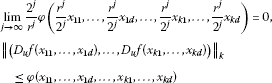(4.7)

for all $u∈U(A)$ and all $x 11 ,…, x 1 d ,…, x k 1 ,…, x k d ∈X$. If there exists an $L<1$ such that

$φ ( r 2 x 11 , r 2 x 11 , … , 0 ⏞ d , r 2 x 21 , r 2 x 21 , … , 0 ⏞ d , … , r 2 x k 1 , r 2 x k 1 , … , 0 ⏞ d ) ≤ r 2 L φ ( x 11 , x 11 , … , 0 ⏞ d , x 21 , x 21 , … , 0 ⏞ d , … , x k 1 , x k 1 , … , 0 ⏞ d )$

for all $x 11 , x 21 ,…, x k 1 ∈X$. Then there exists a unique $A$-linear generalized additive mapping $L:X→Y$ such that

$sup k ∈ N ∥ ( L ( x 1 ) − f ( x 1 ) , … , L ( x k ) − f ( x k ) ) ∥ k ≤ sup k ∈ N L 2 ( d − 2 C l − d − 2 C l − 2 + 1 ) ( 1 − L ) φ ( x 1 , x 1 , 0 , … , 0 ⏞ d − 2 times , … , x k , x k , 0 , … , 0 ⏞ d − 2 times )$

for all $x 1 ,…, x k ∈X$.

Proof Note that $f(0)=0$ and $f(−x)=−f(x)$ for all $x∈X$ since f is an odd mapping. Let $u=1∈U(A)$. Putting $x i 1 = x i 2 = x 1$ and $x i 3 =⋯= x i m =0$, $1≤i≤k$ in (4.7), we have

$∥ ( r f ( 2 r x 1 ) − 2 f ( x 1 ) , … , r f ( 2 r x k ) − 2 f ( x k ) ) ∥ k ≤ 1 C l d − 2 − d − 2 C l − 2 + 1 φ ( x 1 , x 1 , 0 , … , 0 ⏞ d − 2 times , … , x k , x k , 0 , … , 0 ⏞ d − 2 times ) .$

Letting $t: = d − 2 C l − d − 2 C l − 2 +1$, we get

$∥ ( f ( x 1 ) − 2 r f ( r 2 x 1 ) , … , f ( x k ) − 2 r f ( r 2 x k ) ) ∥ k ≤ 1 r t φ ( r 2 x 1 , r 2 x 1 , 0 , … , 0 ⏞ d − 2 times , … , r 2 x k , r 2 x k , 0 , … , 0 ⏞ d − 2 times ) ≤ L 2 t φ ( x 1 , x 1 , 0 , … , 0 ⏞ d − 2 times , … , x k , x k , 0 , … , 0 ⏞ d − 2 times )$

for all $x 1 ,…, x k ∈X$.

The rest of the proof is similar to the proof of Theorem 4.3. □

Corollary 4.6 Let $r<2$, and let θ and $p>1$ be positive real numbers, or let $r>2$, and let θ and $p<1$ be positive real numbers. Let $f:X→Y$ be an odd mapping such that

$∥ ( D u f ( x 11 , … , x 1 d ) , … , D u f ( x k 1 , … , x k d ) ) ∥ k ≤θ ( ∑ j = 1 d ∥ x 1 j ∥ p + ⋯ + ∑ j = 1 d ∥ x k j ∥ p )$

for all $u∈U(A)$ and all $x 11 ,…, x 1 d ,…, x k 1 ,…, x k d ∈X$. Then there exists a unique $A$-linear generalized additive mapping $L:X→Y$ such that

$sup k ∈ N ∥ ( L ( x 1 ) − f ( x 1 ) , … , L ( x k ) − f ( x k ) ) ∥ k ≤ sup k ∈ N r p − 1 θ ( 2 p − 1 − r p − 1 ) ( d − 2 C l − d − 2 C l − 2 + 1 ) ( ∥ x 1 ∥ p + ⋯ + ∥ x k ∥ p )$

for all $x∈X$.

Proof Define

$φ( x 11 ,…, x 1 d ,…, x k 1 ,…, x k d )=θ ( ∑ j = 1 d ∥ x 1 j ∥ p + ⋯ + ∑ j = 1 d ∥ x k j ∥ p ) .$

Putting $L= r p − 1 2 p − 1$ in Theorem 4.5, we get the desired result. □

Now we investigate the Hyers-Ulam stability of linear mappings for the case $d=2$.

Theorem 4.7 Let $r≠2$. Let $f:X→Y$ be an odd mapping for which there is a function $φ: X 2 k →[0,∞)$ such that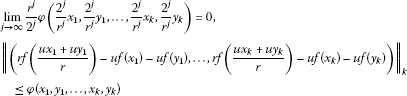(4.8)

for all $u∈U(A)$ and all $x 1 ,… x k , y 1 …, y k ∈X$. If there exists an $L<1$ such that

$φ ( 2 r x 1 , 2 r x 1 , 2 r x 2 , 2 r x 2 , … , 2 r x k , 2 r x k ) ≤ 2 r Lφ( x 1 , x 1 , x 2 , x 2 ,…, x k , x k )$

for all $x 1 ,…, x k ∈X$. Then there exists a unique $A$-linear generalized additive mapping $L:X→Y$ such that

$sup k ∈ N ∥ ( L ( x 1 ) − f ( x 1 ) , … , L ( x k ) − f ( x k ) ) ∥ k ≤ sup k ∈ N L 2 ( 1 − L ) φ ( x 1 , x 1 , … , x k , x k )$

for all $x 1 ,…, x k ∈X$.

Proof Let $u=1∈U(A)$. Putting $x=y$ in (4.8), we have

$∥ ( r f ( 2 r x 1 ) − 2 f ( x 1 ) , … , r f ( 2 r x k ) − 2 f ( x k ) ) ∥ k ≤φ( x 1 , x 1 ,…, x k , x k )$

for all $x∈X$. So

$∥ ( f ( x 1 ) − r 2 f ( 2 r x 1 ) , … , f ( x k ) − r 2 f ( 2 r x k ) ) ∥ k ≤ 1 2 φ( x 1 , x 1 ,…, x k , x k )$

for all $x∈X$.

The rest of the proof is the same as in the proof of Theorem 4.3. □

Corollary 4.8 Let $r>2$, and let θ and $p>1$ be positive real numbers, or let $r<2$, and let θ and $p<1$ be positive real numbers. Let $f:X→Y$ be an odd mapping such that

$∥ ( r f ( u x 1 + u y 1 r ) − u f ( x 1 ) − u f ( y 1 ) , … , r f ( u x k + u y k r ) − u f ( x k ) − u f ( y k ) ) ∥ k ≤ θ ∑ j = 1 k ( ∥ x j ∥ p + ∥ y j ∥ p )$

for all $u∈U(A)$ and for all $x 1 ,…, x k ∈X$. Then there exists a unique $A$-linear generalized additive mapping $L:X→Y$ such that

$sup k ∈ N ∥ ( L ( x 1 ) − f ( x 1 ) , … , L ( x k ) − f ( x k ) ) ∥ k ≤ sup k ∈ N r p − 1 θ r p − 1 − 2 p − 1 ∑ j = 1 k ∥ x j ∥ p$

for all $x 1 ,…, x k ∈X$.

Proof Define $φ( x 1 , y 1 ,…, x k , y k )=θ ∑ j = 1 k ( ∥ x j ∥ p + ∥ y j ∥ p )$, and apply Theorem 4.7. Then we get the desired result. □

Theorem 4.9 Let $r≠2$. Let $f:X→Y$ be an odd mapping for which there is a function $φ: X 2 k →[0,∞)$ such that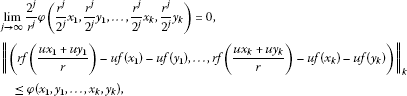(4.9)

for all $u∈U(A)$ and all $x 1 ,…, x k , y 1 ,…, y k ∈X$. If there exists an $L<1$ such that

$φ ( r 2 x 1 , r 2 x 1 , r 2 x 2 , r 2 x 2 , … , r 2 x k , r 2 x k ) ≤ r 2 Lφ( x 1 , x 1 , x 2 , x 2 ,…, x k , x k )$

for all $x 1 ,…, x k ∈X$. Then there exists a unique $A$-linear generalized additive mapping $L:X→Y$ such that

$sup k ∈ N ∥ ( L ( x 1 ) − f ( x 1 ) , … , L ( x k ) − f ( x k ) ) ∥ k ≤ sup k ∈ N 1 2 ( 1 − L ) φ( x 1 , x 1 ,…, x k , x k )$

for all $x 1 ,…, x k ∈X$.

Proof Let $u=1∈U(A)$. Putting $x=y$ in (4.9), we have

$∥ ( r f ( 2 r x 1 ) − 2 f ( x 1 ) , … , r f ( 2 r x k ) − 2 f ( x k ) ) ∥ k ≤φ( x 1 , x 1 ,…, x k , x k )$

for all $x 1 ,…, x k ∈X$. So

$∥ ( f ( x 1 ) − 2 r f ( r 2 x 1 ) , … , f ( x k ) − 2 r f ( r 2 x k ) ) ∥ k ≤ 1 r φ ( r 2 x 1 , r 2 x 1 , … , r 2 x k , r 2 x k ) ≤ 1 2 L φ ( x 1 , x 1 , … , x k , x k )$

for all $x 1 ,…, x k ∈X$.

The rest of the proof is similar to the proof of Theorem 4.3. □

Corollary 4.10 Let $r>2$, and let θ and $p>1$ be positive real numbers. Or let $r<2$, and let θ and $p<1$ be positive real numbers. Let $f:X→Y$ be an odd mapping such that

$∥ ( r f ( u x 1 + u y 1 r ) − u f ( x 1 ) − u f ( y 1 ) , … , r f ( u x k + u y k r ) − u f ( x k ) − u f ( y k ) ) ∥ k ≤ θ ∑ j = 1 k ( ∥ x j ∥ p + ∥ y j ∥ p )$

for all $u∈U(A)$ and all $x 1 ,…, x k ∈X$. Then there exists a unique $A$-linear generalized additive mapping $L:X→Y$ such that

$sup k ∈ N ∥ ( L ( x 1 ) − f ( x 1 ) , … , L ( x k ) − f ( x k ) ) ∥ k ≤ sup k ∈ N r p − 1 θ 2 p − 1 − r p − 1 ∑ j = 1 k ∥ x j ∥ p$

for all $x 1 ,…, x k ∈X$.

Proof Define $φ( x 1 , y 1 ,…, x k , y k )=θ ∑ j = 1 k ( ∥ x j ∥ p + ∥ y j ∥ p )$, and apply Theorem 4.9. Then we get the desired result. □

## 5 Isomorphisms in unital multi-$C ∗$-algebras

Throughout this section, assume that $A$ and $B$ are unital multi-$C ∗$-algebras with unit e. Let $U(A)$ be the set of unitary elements in $A$.

We investigate $C ∗$-algebra isomorphisms in unital multi-$C ∗$-algebras.

Theorem 5.1 Let $r≠2$. Let $h:A→B$ be an odd bijective mapping satisfying $h( 2 n r n uy)=h( 2 n r n u)h(y)$ for all $u∈U(A)$, all $y∈A$, and $n=0,1,2,…$, for which there exists a function $φ: A k d →[0,∞)$ such that

$lim j → ∞ r j 2 j φ ( 2 j r j x 11 , … , 2 j r j x 1 d , … , 2 j r j x k 1 , … , 2 j r j x k d ) = 0 , ∥ ( D μ h ( x 11 , … , x 1 d ) , … , D μ h ( x k 1 , … , x k d ) ) ∥ k ≤ φ ( x 11 , … , x 1 d , … , x k 1 , … , x k d ) , ∥ ( h ( 2 n r n u 1 ∗ ) − h ( 2 n r n u 1 ) ∗ , … , h ( 2 n r n u k ∗ ) − h ( 2 n r n u k ) ∗ ) ∥ k ≤ φ ( 2 n r n u 1 , … , 2 n r n u 1 ⏞ d times , … , 2 n r n u k , … , 2 n r n u k ⏞ d times )$

for all $μ∈ S 1 :={λ∈C∣|λ|=1}$, all $u 1 ,…, u k ∈U(A)$, $n=0,1,2,…$, and all $x 11 ,…, x k d ∈A$. Assume that $lim n → ∞ r n 2 n h( 2 n r n e)$ is invertible. Then the odd bijective mapping $h:A→B$ is a $C ∗$-algebra isomorphism.

Proof Consider the multi-$C ∗$-algebras $A$ and $B$ as left Banach modules over the unital multi-$C ∗$-algebra $C$. By Theorem 4.3, there exists a unique $C$-linear generalized additive mapping $H:A→B$ such that

$sup k ∈ N ∥ ( h ( x 1 ) − H ( x 1 ) , … , h ( x k ) − H ( x k ) ∥ k ≤ sup k ∈ N 1 2 ( d − 2 C l − d − 2 C l − 2 + 1 ) φ ( x 1 , x 1 , 0 , … , 0 ⏞ d − 2 times , … , x k , x k , 0 , … , 0 ⏞ d − 2 times )$

for all $x 1 ,…, x k ∈A$ in which $H:A→B$ is given by

$H(x)= lim n → ∞ r n 2 n h ( 2 n r n x )$

for all $x∈A$.

The rest of the proof is similar to the proof of Theorem 4.1 of . □

Corollary 5.2 Let $r>2$, and let θ and $p>1$ be positive real numbers. Or let $r<2$, and let θ and $p<1$ be positive real numbers. Let $h:A→B$ be an odd bijective mapping satisfying $h( 2 n r n uy)=h( 2 n r n u)h(y)$ for all $u∈U(A)$, all $y∈A$, and all $n=0,1,2,…$, such that

$∥ ( D μ h ( x 11 , … , x 1 d ) , … , D μ h ( x k 1 , … , x k d ) ) ∥ k ≤ θ ∑ j = 1 d ( ∥ x 1 j ∥ p + ⋯ + ∥ x k j ∥ p ) , ∥ ( h ( 2 n r n u 1 ∗ ) − h ( 2 n r n u 1 ) ∗ , … , h ( 2 n r n u k ∗ ) − h ( 2 n r n u k ) ∗ ) ∥ k ≤ k d 2 p n r p n θ$

for all $μ∈ S 1$, all $u∈U(A)$, $n=0,1,2,…$, and all $x 11 ,…, x k d ∈A$. Assume that $lim n → ∞ r n 2 n h( 2 n r n e)$ is invertible. Then the odd bijective mapping $h:A→B$ is a $C ∗$-algebra isomorphism.

Proof Define $φ( x 11 ,…, x 1 d ,…, x k 1 ,…, x k d )=θ ∑ j = 1 d ( ∥ x 1 j ∥ p +⋯+ ∥ x k j ∥ p )$, and apply Theorem 5.1. Then we get the desired result. □

## References

1. 1.

Rassias TM: On the stability of the linear mapping in Banach spaces. Proc. Am. Math. Soc. 1978, 72: 297–300. 10.1090/S0002-9939-1978-0507327-1

2. 2.

Găvruta P: A generalization of the Hyers-Ulam-Rassias stability of approximately additive mappings. J. Math. Anal. Appl. 1994, 184: 431–436. 10.1006/jmaa.1994.1211

3. 3.

Park C: On the stability of the linear mapping in Banach modules. J. Math. Anal. Appl. 2002, 275: 711–720. 10.1016/S0022-247X(02)00386-4

4. 4.

Rassias TM: On the stability of functional equations and a problem of Ulam. Acta Appl. Math. 2000, 62: 23–130. 10.1023/A:1006499223572

5. 5.

Eshaghi Gordji M, Khodaei H: Stability of Functional Equations. Lap Lambert Academic Publishing, Saarbrücken; 2010.

6. 6.

Jung S-M Springer Optimization and Its Applications 48. In Hyers-Ulam-Rassias Stability of Functional Equations in Nonlinear Analysis. Springer, New York; 2011.

7. 7.

Baak C, Boo D, Rassias TM:Generalized additive mapping in Banach modules and isomorphisms between $C ∗$-algebras. J. Math. Anal. Appl. 2006, 314: 150–156. 10.1016/j.jmaa.2005.03.099

8. 8.

Cădariu L, Radu V: Fixed points and the stability of Jensen’s functional equation. J. Inequal. Pure Appl. Math. 2003., 4: Article ID 4

9. 9.

Radu V: The fixed point alternative and the stability of functional equations. Fixed Point Theory 2003, 4: 91–96.

10. 10.

Diaz J, Margolis B: A fixed point theorem of the alternative for contractions on a generalized complete metric space. Bull. Am. Math. Soc. 1968, 74: 305–309. 10.1090/S0002-9904-1968-11933-0

11. 11.

Dales, HG, Polyakov, ME: Multi-normed spaces and multi-Banach algebras. Preprint

12. 12.

Dales HG, Moslehian MS: Stability of mappings on multi-normed spaces. Glasg. Math. J. 2007, 49: 321–332. 10.1017/S0017089507003552

13. 13.

Moslehian MS, Nikodem K, Popa D: Asymptotic aspect of the quadratic functional equation in multi-normed spaces. J. Math. Anal. Appl. 2009, 355: 717–724. 10.1016/j.jmaa.2009.02.017

14. 14.

Moslehian MS: Superstability of higher derivations in multi-Banach algebras. Tamsui Oxf. J. Math. Sci. 2008, 24: 417–427.

15. 15.

Dales HG London Mathematical Society Monographs. New Series 24. In Banach Algebras and Automatic Continuity. Oxford University Press, Oxford; 2000.

## Acknowledgements

The authors are grateful to the reviewers for their valuable comments and suggestions.

## Author information

Authors

### Competing interests

The authors declare that they have no competing interests.

### Authors’ contributions

All authors carried out the proof. All authors conceived of the study and participated in its design and coordination. All authors read and approved the final manuscript.

## Rights and permissions

Reprints and Permissions

Park, C., Saadati, R. Approximation of a generalized additive mapping in multi-Banach modules and isomorphisms in multi-$C ∗$-algebras: a fixed-point approach. Adv Differ Equ 2012, 162 (2012). https://doi.org/10.1186/1687-1847-2012-162

• $C ∗$-algebra isomorphism
• multi-Banach module over multi-$C ∗$-algebra